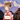Home > Lessons > Complete Beginner's Guide To Conjugating Verbs In Japanese

# Complete Beginner's Guide To Conjugating Verbs In Japanese

Definition: In Japanese the conjugation of verbs is more complicated than English, because in Japanese the conjugation in future and present is same but exit the past, negative, form i, form te, Potential, Causative, Passive causative, Imperative, Passive, Volitional, form conditionally eba, form conditionally ra.

## Conjugation in Present and Future

In Japanese the present and future are same and the difference the put the context of time in where you have the talk, in these tenses is not necessary the conjugation.

Example:

• Present: I eat fish = = Watashi wa sakana o taberu

• Future: I eat fish = = Watashi wa sakana o taberu

## Conjugation in Past

### For Irregular Verbs

For make this conjugation, you should do the replacement of the letters you see to below, this letters you find in finish the verbs or adjectives.

 **Present** **Past** す る = suru し た = shita 来 る = kuru 来 た = kita 行 く = iku 行 っ た = itta い ら っ し ゃ る = irassharu い ら っ し ゃ っ た = irasshatta ま す = masu ま し た = mashita

### For Regular VerbsFor make this conjugation, you should do the replacement of the letters you see to below, this letters you find in finish the verbs or adjectives.

 **Present** **Past** う = u っ た = tta く = ku い た = ita ぐ = gu い だ ida す = su し た = shita つ = tsu っ た = tta ぬ = nu ん だ = nda ぶ = bu ん だ = nda む = mu ん だ = nda る = ru っ た = tta い る = iru い た = ita え る = eru え た = eta

Examples:

• 使 = tsukau (Present)

• 使 = tsukatta (Past)

• = oyogu* (Present)

• = oyoida* (Past)

• = matsu* (Present)

• = matta* (Past)

• = yobu* (Present)

• = yonda* (Past)

• = kigaeru* (Present)

• = kigaeta* (Past)

### For AdjectivesFor make this conjugation, you should do the replacement of the letters you see to below, this letters you find in finish the verbs or adjectives.

 **Present** **Past** い = i か っ た = katta な = na だ っ た = datta

Examples:

• yasui*

• yasukatta*

• kantan*

• kantan datta*

## Conjugation in Negative

The basic pattern is to convert u in nai or anai.

### For Irregular Verbs

 **Infinitive** **Negative** す る = suru し な い = shinai 来 る = kuru 来 な い = konai あ る = aru な い = nai だ = da じ ゃ な い = ja nai ま す = masu ま せ ん = masen

Examples:

• = benkyō suru*

• = benkyō shinai*

• = ikimasu*

• = ikimasen*

### For Regular Verbs

 **Infinitive** **Negative** う = u わない = wanai く = ku かない = kanai ぐ = gu がない = ganai す = su さない = sanai つ = tsu たない = tanai ぬ = nu なない = nanai ぶ = bu ばない = banai む = mu まない = manai る = ru らない = ranai い る = iru い な い = inai え る = eru え な い = enai

Examples:

• = matsu*

• = matanai*

• = shinu*

• = shinanai*

• = hashiru*

• = hashiranai*

 **Present** **Past** い = i く な い = kunai な = na じ ゃ な い = ja nai

Examples:

• itai*

• itakunai*

• kantan*

• kantan ja nai*##### Author:Ichika
"新しい言語は、新たな人生の始まり。"
Learn Japanese here.

Make sure to subscribe.